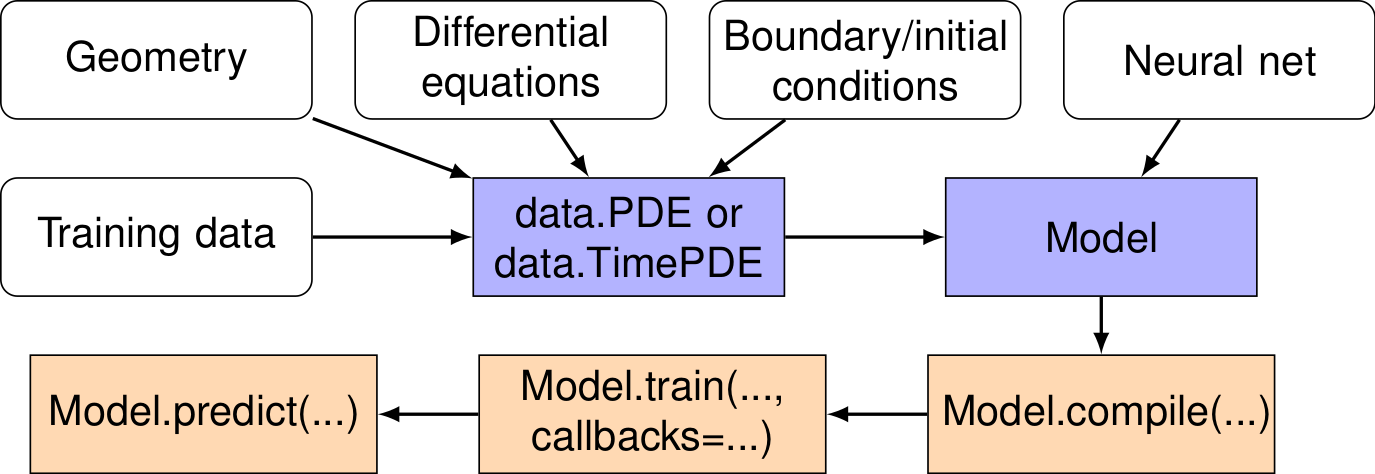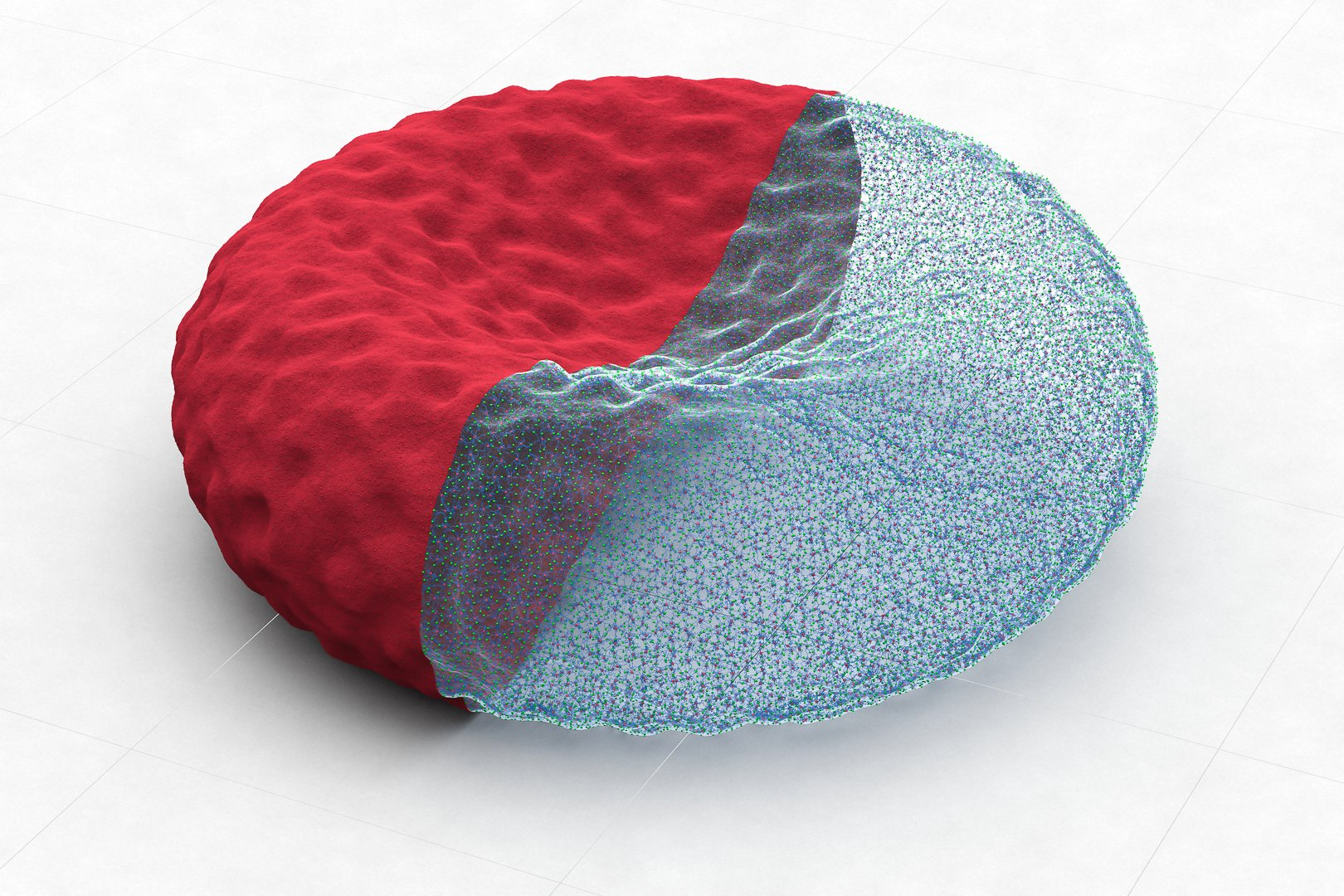# Softwares

## DeepXDEsource

DeepXDE is a deep learning library for solving differential equations on top of TensorFlow.

Use DeepXDE if you need a deep learning library that

• solves partial differential equations (PDEs),
• solves integro-differential equations (IDEs),
• solves fractional partial differential equations (fPDEs),
• solves inverse problems for differential equations,
• approximates functions from a dataset with/without constraints,
• approximates functions from multi-fidelity data.

DeepXDE is extensible to solve other problems in scientific computing.## OpenRBCsource

OpenRBC is a coarse-grained molecular dynamics code for simulating entire human red blood cells at the protein resolution. It is one of the winners in the IBM OpenPower Developer Challenge competition. The code outperforms a legacy solver by 10 times in time-to-solution through the use of an novel adaptive spatial searching algorithm to accelerate the computation of short-range pairwise interactions in an extremely sparse 3D space.